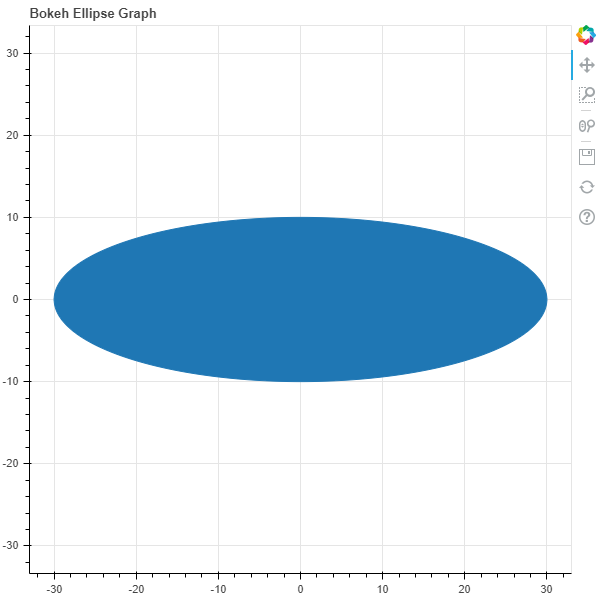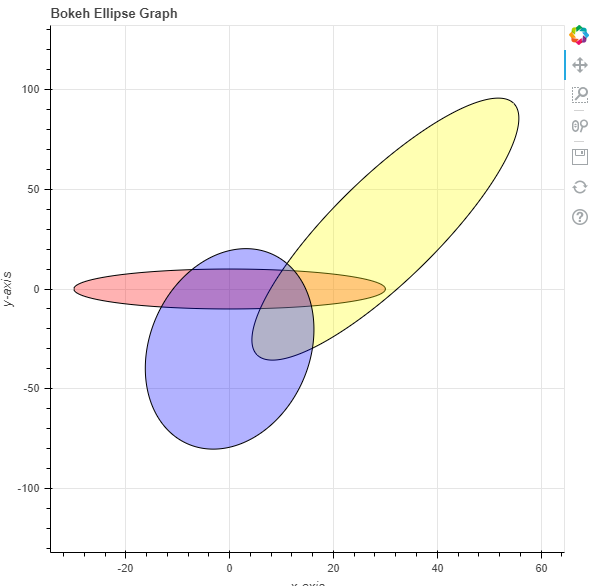GeeksforGeeks App
Open AppBrowser
Continue

# Python Bokeh – Plotting Ellipses on a Graph

Bokeh is a Python interactive data visualization. It renders its plots using HTML and JavaScript. It targets modern web browsers for presentation providing elegant, concise construction of novel graphics with high-performance interactivity.

Bokeh can be used to plot ellipses on a graph. Plotting ellipses on a graph can be done using the `ellipse()` method of the `plotting` module.

## plotting.figure.ellipse()

Syntax : ellipse(parameters)

Parameters :

• x : x-coordinates of the center of the ellipse
• y : y-coordinates of the center of the ellipse
• width : width of each ellipse
• height : height of each ellipse
• angle : angle of rotation of the ellipse, default is 0
• angle_units : unit of the angle, default is rad
• fill_alpha : fill alpha value of the ellipse
• fill_color : fill color value of the ellipse
• height_units : unit of the height, default isdata
• line_alpha : percentage value of line alpha, default is 1
• line_cap : value of line cap for the line, default is butt
• line_color : color of the line, default is black
• line_dash : value of line dash such as :
• solid
• dashed
• dotted
• dotdash
• dashdot

default is solid

• line_dash_offset : value of line dash offset, default is 0
• line_join : value of line join, default in bevel
• line_width : value of the width of the line, default is 1
• name : user-supplied name for the model
• tags : user-supplied values for the model

Other Parameters :

• alpha : sets all alpha keyword arguments at once
• color : sets all color keyword arguments at once
• legend_field : name of a column in the data source that should be used
• legend_group : name of a column in the data source that should be used
• legend_label : labels the legend entry
• muted : determines whether the glyph should be rendered as muted or not, default is False
• name : optional user-supplied name to attach to the renderer
• source : user-supplied data source
• view : view for filtering the data source
• visible : determines whether the glyph should be rendered or not, default is True
• x_range_name : name of an extra range to use for mapping x-coordinates
• y_range_name : name of an extra range to use for mapping y-coordinates
• level : specifies the render level order for this glyph

Returns : an object of class `GlyphRenderer`

Example 1 :In this example we will be using the default values for plotting the graph.

 `# importing the modules``from` `bokeh.plotting ``import` `figure, output_file, show``     ` `# file to save the model``output_file(``"gfg.html"``)``     ` `# instantiating the figure object``graph ``=` `figure(title ``=` `"Bokeh Ellipse Graph"``,``               ``match_aspect ``=` `True``)``   ` `# the points to be plotted``x ``=` `0``y ``=` `0`` ` `# width of the ellipse``width ``=` `60`` ` `# height of the ellipse``height ``=` `20`` ` `# plotting the graph``graph.ellipse(x, y,``              ``width ``=` `width,``              ``height ``=` `height)``  ` `# displaying the model``show(graph)`

Output :Example 2 :In this example we will be plotting multiple ellipses with different parameters.

 `# importing the modules``from` `bokeh.plotting ``import` `figure, output_file, show``     ` `# file to save the model``output_file(``"gfg.html"``)``     ` `# instantiating the figure object``graph ``=` `figure(title ``=` `"Bokeh Ellipse Graph"``)`` ` `# name of the x-axis``graph.xaxis.axis_label ``=` `"x-axis"``    ` `# name of the y-axis``graph.yaxis.axis_label ``=` `"y-axis"``   ` `# the points to be plotted``x ``=` `[``0``, ``30``, ``0``]``y ``=` `[``0``, ``30``, ``-``30``]`` ` `# width of the ellipse``width ``=` `[``60``, ``20``, ``40``]`` ` `# height of the ellipse``height ``=` `[``20``, ``180``, ``80``]`` ` `# angle of the ellipse``angle ``=` `[``0``, ``150``, ``20``]`` ` `# fill alpha values``fill_alpha ``=` `0.3`` ` `# fill color values``fill_color ``=` `[``"red"``, ``"yellow"``, ``"blue"``]`` ` `# line color value``line_color ``=` `"black"`` ` `# plotting the graph``graph.ellipse(x, y,``              ``width ``=` `width,``              ``height ``=` `height,``              ``angle ``=` `angle,``              ``fill_alpha ``=` `fill_alpha,``              ``fill_color ``=` `fill_color,``              ``line_color ``=` `line_color)``  ` `# displaying the model``show(graph)`

Output :My Personal Notes arrow_drop_up